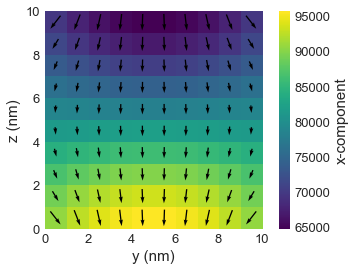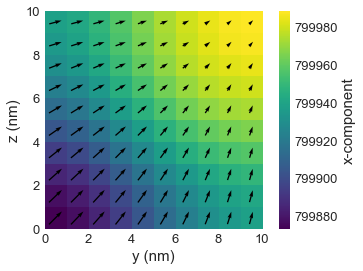# Tutorial 11: Deriving energy values¶

Interactive online tutorial:In this tutorial, we show how derived fields and values can be computed afetr the micromagnetic system is defined.

## Simulation¶

First of all, as usual, we import oommfc and discretisedfield.

In :
import oommfc as oc
import discretisedfield as df
import micromagneticmodel as mm
%matplotlib inline


We define the cube mesh with edge length $10 \,\text{nm}$ and cell discretisation edge $1 \,\text{nm}$.

In :
mesh = df.Mesh(p1=(0, 0, 0), p2=(10e-9, 10e-9, 10e-9), cell=(1e-9, 1e-9, 1e-9))
mesh.k3d()


Now we define the system object and its Hamiltonian.

In :
system = mm.System(name='system')

A = 1e-11
H = (0.1/mm.consts.mu0, 0, 0)
K = 1e3
u = (1, 1, 1)
system.energy = mm.Exchange(A=A) + \
mm.Demag() + \
mm.Zeeman(H=H) + \
mm.UniaxialAnisotropy(K=K, u=u)


We will now intialise the system in $(0, 0, 1)$ direction and relax the magnetisation.

In :
Ms = 8e5
system.m = df.Field(mesh, dim=3, value=(0, 0, 1), norm=Ms)


## Effective field¶

Total effective field is:

In :
oc.compute(system.energy.effective_field, system).plane('x').mpl()

Running OOMMF (ExeOOMMFRunner) [2020/06/12 00:54]... (2.2 s)Whereas, the individual exchange effective field is:

In :
Hex_eff = oc.compute(system.energy.exchange.effective_field, system)

Running OOMMF (ExeOOMMFRunner) [2020/06/12 00:54]... (1.8 s)


Because we initialised the system with the uniform state, we expect this effective field to be zero.

In :
Hex_eff.average

Out:
(0.0, 0.0, 0.0)

## Relax the system¶

In :
md = oc.MinDriver()
md.drive(system)

Running OOMMF (ExeOOMMFRunner) [2020/06/12 00:54]... (1.9 s)


Compute the energy (and demonstrate that the energy decreased) and plot its magnetisation:

In :
E = oc.compute(system.energy.energy, system)
print("The system's energy is {} J.".format(E))

system.m.plane('x').mpl()

Running OOMMF (ExeOOMMFRunner) [2020/06/12 00:54]... (1.8 s)
The system's energy is 5.35285533145e-20 J.## Computing energies of individual term¶

For instance, the exchange energy is:

In :
oc.compute(system.energy.exchange.energy, system)

Running OOMMF (ExeOOMMFRunner) [2020/06/12 00:54]... (1.9 s)

Out:
1.12170206436e-21

We can also chack the sum of all individual energy terms and check if it the same as the total energy.

In :
total_energy = 0
for term in system.energy:
total_energy += oc.compute(term.energy, system)

print("The sum of energy terms is {} J.".format(total_energy))
print("The system's energy is {} J.".format(oc.compute(system.energy.energy, system)))

Running OOMMF (ExeOOMMFRunner) [2020/06/12 00:54]... (1.8 s)
Running OOMMF (ExeOOMMFRunner) [2020/06/12 00:54]... (1.7 s)
Running OOMMF (ExeOOMMFRunner) [2020/06/12 00:54]... (1.7 s)
Running OOMMF (ExeOOMMFRunner) [2020/06/12 00:54]... (1.8 s)
The sum of energy terms is 5.352855331433802e-20 J.
Running OOMMF (ExeOOMMFRunner) [2020/06/12 00:54]... (1.8 s)
The system's energy is 5.35285533145e-20 J.